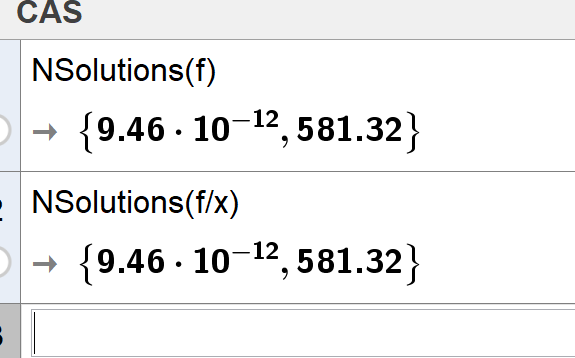# Dynamic sliders

NUMB3RS shared this question 2 years ago

Hi, could you help me with this? . . . I thought I knew it but I don't remember how I did it before.I would like to set the minimum of the slider "t" equal zero and the maximum to be x(C). Where C is the point where the curve cuts the x-axis, but it isn't working. Why is the slider ignoring the max value?

Thank you1

I mean

C is OK

t is OK

but a is wrong

Maybe instead of a you are looking for something like the following:

ft(x) = If(0 < x < t, f(x))1

Thank you very much rami! . . . that solved my problem :)1

Hi Rami, I'm having problem with this applet which is a variation of the one that you help me before. I would like to know why the x-value of point B (intersection of the function f and the x-axis) becomes undefined for certain values of k, m and theta?. The maximum value for the slider t is tt and it depends of B to work. Could you please help me?

Thanks, Jose1

Try NSolutions(f) or NSolutions(f/x) to remove the x=0 root. You may still get some spurious answers to get rid of:1

This version (correction of object B) should work.1

Thank you Michael and Rami . . . it's so good that you have not only the knowledge but you take the time to help others. That's highly appreciated. Thanks for solving my problems

Jose